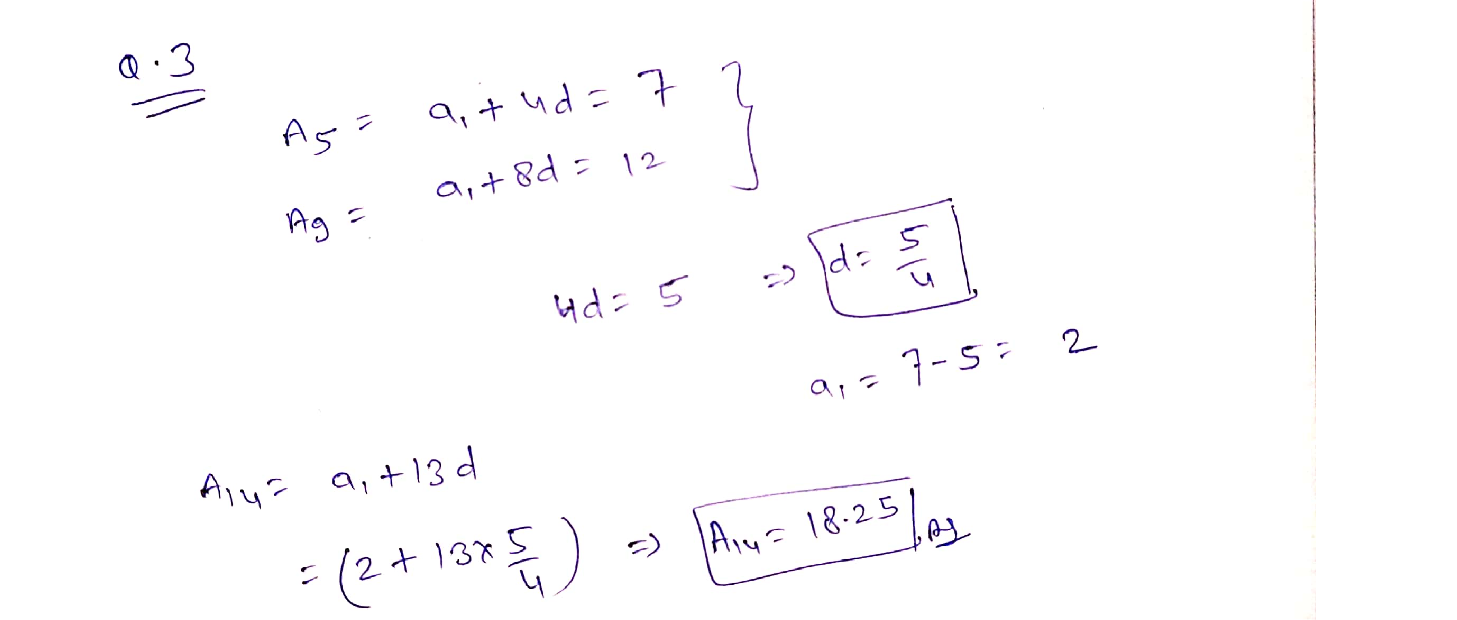Question

Differential Equations

3. The fifth term of an arithmetic progression is 7 and the ninth term is 12. Calculate the fourteenth term.Verified### Question 44953Differential Equations

Consider the IVP y = x + 2y, y(0) = 1
A) (8 pts) Use Euler's method to obtain an approximation of y(0.5) usingh = 0.25 for the given IVP (Use four-decimal approximation)
B) (12 pts) Use Euler's method to obtain an approximation of y(0.5) usingh = 0.1 for the given IVP (Use four-decimal approximation)
A bacteria culture initially has 100 number of bacteria and doubles in size in 2hours. Assume that the rate of increase of the culture is proportional to the size.
Write the initial value problem for the bacteria culture and solve it
ts) How long will it take for the size to triple?
Verify that y(x) = c1 cos(6x) + c2 sin(6x) is a solution of
y" + 36y = 0
s) Either solve the boundary value problem
y^{\prime \prime}+36 y=0, y(0)=0, y\left(\frac{2 \pi}{6}\right)=1
or else show that it has no solution

### Question 44952Differential Equations

The solution of the problem
y^{\prime}=x+2 y, y(1)=2
numerically using Euler's method for y(1.6) using h = 0.3 is
-5.99
3.5
-3.5
5.99
None of the others

### Question 44951Differential Equations

A differential equation
y^{\prime \prime}-5 y^{\prime}+2 y=0
\text { with } y(0)=1 \text { and } y^{\prime}(0)=5 \text { is }
a second order initial value problem
a fourth order initial value problem
a third order initial value problem
None of the others
A boundary value problem

### Question 44950Differential Equations

The solution of the initial value problem
y^{\prime \prime}+16 y=0, y(0)=0, y^{\prime}(\pi)=4
\sin 4 x
-\sin 4 x
\text { None of the others }
\cos 4 x
\cos 4 x+\sin 4 x

### Question 44949Differential Equations

The population, P, of a town increases as the following equation:
P(t)=P_{0} e^{0.25 t}
If P(5) = 200, what is the initial population?
Select one:
Po - 59
None of the others
Po - 61
Po - 60
Po 57

### Question 44948Differential Equations

For the following model of Newton's law of cooling:
\frac{d T}{d t}=\ln \left(\frac{1}{2}\right)(T-16), \quad T(0)=70
\text { the ambient temperature } T_{m} \text { is } 16 .
True--False

### Question 44947Differential Equations

Consider the initial-value problem
y^{\prime}-20=y e^{2 x}, y(1)=5
Using the Euler's method we have
y_{1}=5+h\left(20+5 e^{2}\right)

### Question 44946Differential Equations

\text { If } P(t)=2 e^{0.15 t} \text { gives the population in an environment at time } t \text {, then }
P(4)=2 e^{0.06}
O TrueO False

### Question 44945Differential Equations

\text { The function } y=e^{-8 x} \text { is a solution of the initial value problem }
y^{\prime \prime}-64 y=0, y(0)=1, y^{\prime}(0)=8 .
True--False

### Question 44710Differential Equations

Q2.[40pt] Draw the phase diagram of the following (system of) differential/difference equa-tions and analyze the stability of the equilibria.
\dot{x}_{t}=\frac{x_{t}}{x_{t}^{2}+1}
\dot{x}_{t}=x_{t} y_{t}
\dot{y}_{t}=-2 x_{t}-4 y_{t}+4
\dot{x}_{t}=3 x_{t}-13 y_{t}
\dot{y}_{t}=5 x_{t}+y_{t}
x_{t+1}=2-x_{t} .

### Submit query

Getting answers to your urgent problems is simple. Submit your query in the given box and get answers Instantly.

### Submit a new QuerySuccess

Assignment is successfully created